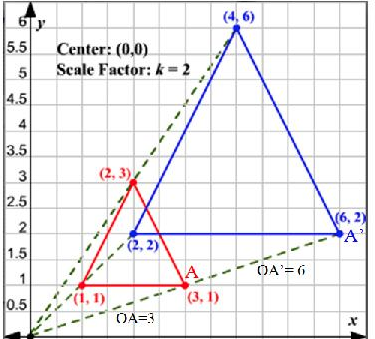Dilations
3 years ago
tinkablue
Save
Edit
Host a game
Live GameLive
Homework
Solo Practice
Practice15 QuestionsShow answers
• Question 1
30 seconds
Q. Dilate the figure by a scale factor of 3 with the origin as the center of dilation. What are the coordinates of the image?
A(3,3) B(6,6) C(9,3)
A'(9,9) B'(18,18) C'(27,9)
A'(6,6) B'(9,9) C'(12,6)
A'(0,0) B'(3,3) C'(6,0)
A'(1,1) B'(2,2) C'(3,1)
• Question 2
60 secondsQ. Tell whether the transformation is a dilation. Explain.
No, scale factor isn't the same.
Yes, scale factor is the same.
• Question 3
120 seconds
Q. A triangle has vertices with coordinates (2,0), (3, -1) and (-2,-5). If the triangle is dilated by a scale factor of 3 with the origin as the center of dilation, what are the coordinates of the vertices of the image?
(5,3), (6,2), (1,-2)
(6,0), (9,-3), (-6,-15)
(2/3,0), (1,-1/3), (-2/3,-5/3)
(-1,-3), (0,-4), (-5,-8)
• Question 4
60 seconds
Q. The __________ is the ratio of a length of the new figure to the corresponding length on the original figure.
reduction
enlargement
scale factor
center of dilation
• Question 5
60 seconds
Q. State the coordinate of the image of the given point B (-10,-6) under a dilation with center at the origin with the given scale factor k = 1/2.
(5,3)
(20,12)
(-20,-12)
(-5,-3)
• Question 6
60 seconds
Q. The point (8, 12) was dilated to become point (2, 3). What was the scale factor?
½
¼
4
2
• Question 7
60 secondsQ. Dilate Point B by a scale factor of 1/2
(1.5,-4)
(-1.5,1)
(-1,-1.5)
(-2,-2)
• Question 8
60 seconds
Q. In a dilation what mathematical operation is used with the scale factor?
subtraction
multiplication
division
• Question 9
30 secondsQ. The red triangle has been dilated by a scale factor of 1/4, which triangle is it's new image?
green triangle
red triangle
black triangle
none of these
• Question 10
30 seconds
Q. A scale factor of less than one means
that the image will be larger than the pre-image.
That the image will be the same as the pre-image.
That you need to subtract that amount off  of each side.
That the image will be a reduction of the pre-image.
• Question 11
30 secondsQ. What is the definition of dilations ,in mathematical terms?
to make larger or smaller
to make larger
all of the above
to make smaller
• Question 12
30 seconds
Q. A dilation can produce a smaller figure or a(n)______
reduction
enlargement
scale factor
center of dilation
• Question 13
30 seconds
Q.  A dilation can produce a larger figure or a(n)______
reduction
enlargement
scale factor
center of dilation
• Question 14
60 secondsQ. Does the image show a Dilation?
Yes
No
• Question 15
30 secondsQ.